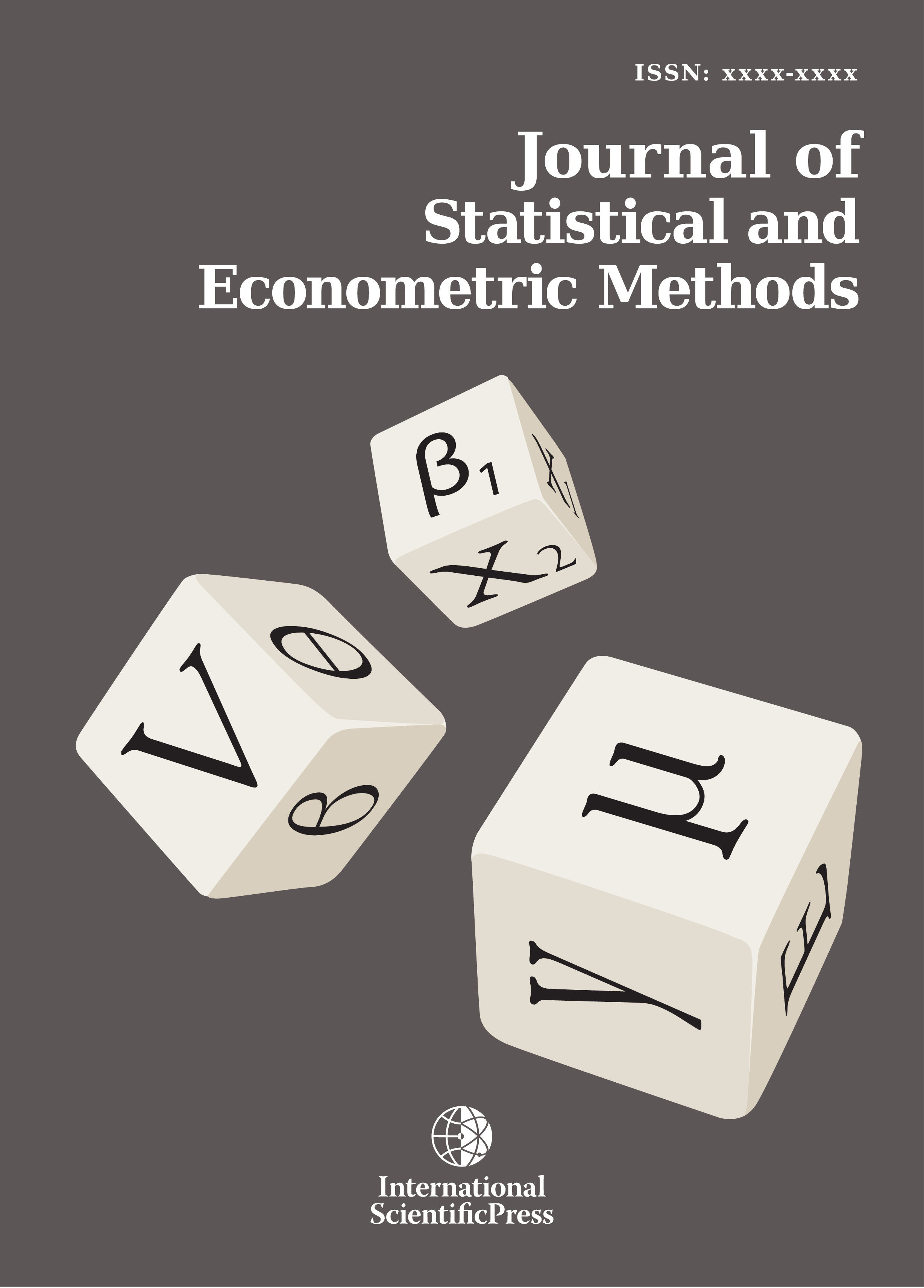# Journal of Statistical and Econometric Methods

#### Time Series Forecasting: A Comparative Study of VAR ANN and SVM Models

•[ Download ]
• Abstract

Modeling and forecasting time series data has primary significance to numerous practical areas. Numerous significant models have been proposed in texts for enlightening the correctness and effectiveness of modeling and predicting time series data. The goal of this work is to discovery an appropriate model to forecast time series data. Firstly, we applied the most popular multivariate time series model is Vector Autoregressive model, with its frequently used four criteria of VAR order selection such as AIC, HQ, SC and FPE,  and the asymptotic Portmanteau test of VAR order selection. We also checked the accuracy of forecast performance based on impulse response function. Secondly, we applied the most popular time series modeling and forecasting machine learning techniques, such as artificial neural network and support vector machine. In this study, we applied the three multivariate time series models, viz. VAR, ANN and SVM, compared together with their inherent forecasting strengths based on the five forecast performance measures: mean squared error, mean absolute deviation, root mean squared error, mean absolute percentage error and Theil’s U-statistics. Finally, we found that the artificial neural network, time series modeling and forecasting machine learning technique, is the best technique for time series modeling and forecasting.

Keywords: Forecasting, VAR, ANN, SVM, Forecast Performance Measures.

Mathematics Subject Classification: 60G10, 60G15, 82C05, 53A17ISSN: 2241-0376 (Online)
2241-0384 (Print)